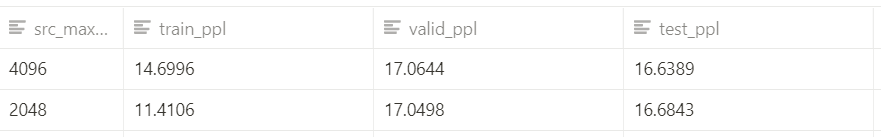# Difference in the length of positional embeddings produce different results

Hi, I am currently experimenting with how the length of dialogue histories in one input affects the performance of dialogue models using multi-session chat data. While I am working on BlenderbotSmallForConditionalGeneration from Huggingface’s transformers with the checkpoint “blenderbot_small-90M”, I encountered results which are not understandable for me.

Since I want to put long inputs(ex. 1024, 2048, 4096…), I expanded the positional embedding matrix of the encoder since it is initialized in the size (512, 512). I copied the first 512 embeddings and appended them repeatedly to make the embedding matrix the size I want. On the other hand, I truncated the position embedding matrix of the decoder into (128, 512), since the max target length is 128.

``````from transformers import BlenderbotSmallForConditionalGeneration
from transformers.models.blenderbot.modeling_blenderbot import BlenderbotLearnedPositionalEmbedding
from torch import nn

import torch

src_max_len = SRC_MAX_LEN  # 4906, 2048, 1024...
trg_max_len = 128

def reset_position_embeddings():
# Expand encoder position embedding.
encoder_weights = model.model.encoder.embed_positions.weight.data
model.model.encoder.embed_positions = BlenderbotLearnedPositionalEmbedding(src_max_len, model.config.d_model)

num_repeats = src_max_len // model.config.max_position_embeddings
new_encoder_weights = encoder_weights.repeat(num_repeats, 1)
model.model.encoder.embed_positions.weight = nn.Parameter(new_encoder_weights)

assert torch.equal(model.model.encoder.embed_positions.weight.data, encoder_weights.repeat(num_repeats, 1))

model.config.max_length = src_max_len
model.config.max_position_embeddings = self.args.src_max_len

# Truncate decoder position embedding.
decoder_weights = model.model.decoder.embed_positions.weight.data
model.model.decoder.embed_positions = BlenderbotLearnedPositionalEmbedding(trg_max_len, model.config.d_model)